Readers new to PRTools should start by reading the PRTools User Guide.

There is a set of introductory examples that may serve as a part of an introductory PRTools course. These are based on code snippets that can be used by copy-paste-run next to a Matlab window,

Below the growing set of advanced PRTools example files is presented. These are published Matlab m-files that can be run by the user. In various documents references to these files are made.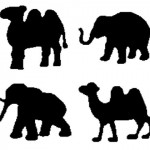A simple Kimia image classification, Introductory PR experiment on blob images. It loads the images, computes features, creates scatter plot and estimates the nearest neighbor classification error.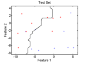Classifiers, Introduction of defining, training and evaluating classifiers.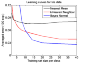Learning curves, Learning curves for Bayes-Normal, Nearest Mean and Nearest Neighbor on the Iris dataset. Averages over 100 repetitions.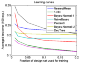Cross-validation, A large experiment comparing the ability of several cross-validation procedures to determine the best of seven classifiers.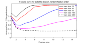Feature curves, Feature curves for Bayes-Normal, on the Satellite dataset.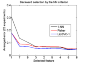Feature selection 1, Examples of various feature selection procedures, organized per procedure.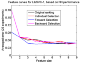Feature selection 2, Examples of various feature selection procedures, organized per classifier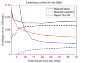The apparent error, Examples of the behavior of the apparent error for increasing training set size, dimensionality and complexity.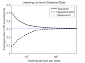Bayes classifier uses a 2D examples of four normally distributed classes to show the Bayes classifier and how it can be approximated by qdc. A learning curve shows the speed of convergence to the Bayes error.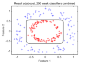Adaboost introduction, Adaboost 2D examples based on perceptrons and decision stumps.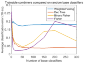Adaboost comparison, Adaboost compared with other base classifier generators and combiners.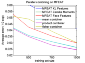Combining classifiers, Introduction of stacked and parallel combining by fixed and trained combiners.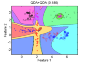Multi-class classifiers, improved by using a trained combiner for post-processing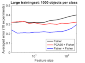PCA versus classifier for feature reduction. An example comparing by feature curves the performance of PCA with that of a trained classifier for feature reduction in a multi-class problem.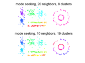Clustering, Introduction of various clustering techniques.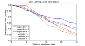Semi-supervised learning by PCA, Illustrates that semi-supervised classification by PCA may be useful.